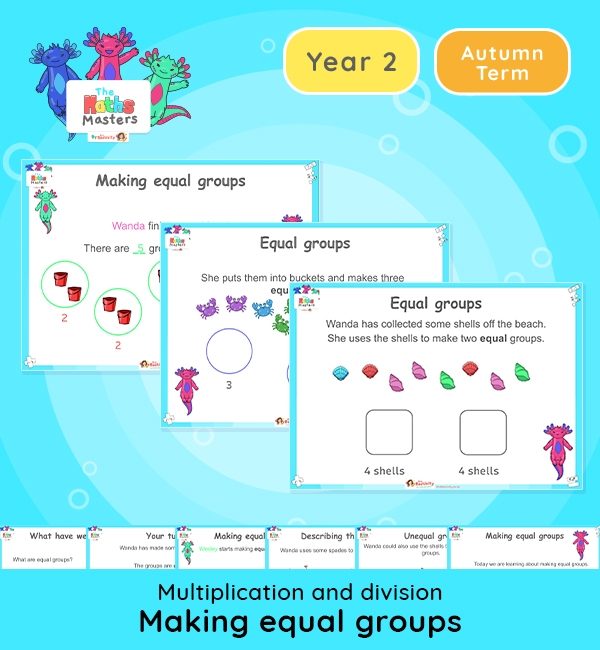# Year 2 | Making Equal Groups Lesson Presentation## Year 2 multiplication and division resources

Aligned with the maths mastery approach, this Year 2 | Making Equal Groups Lesson Presentation is fully editable, and is designed for the Year 2 maths curriculum covering the following maths objectives for the autumn term:

Topic: Multiplication and division

This is lesson focuses on making equal groups and reviews some of the learning from Year 1. You may wish to split into two lessons and share the content over two sessions.

Small Steps: Make equal groups

NC Links: Recall and use multiplication and division facts for the 2,5, and 10 times tables. *solve problems involving multiplication and division using materials, array, repeated addition, mental methods and multiplication and division facts including problems in context.

TAF Statements:– Recall and use multiplication and division facts for 2, 5, and 10 and use them to solve simple problems. Working At

Greater Depth – Use reasoning about number and relationships to solve more complex problems and explain their thinking.

Year 1 conceptual prerequisites: Count in multiples of 2, 5 and 10

Explore our other year 2 multiplication and division resources.

## Recently Viewed